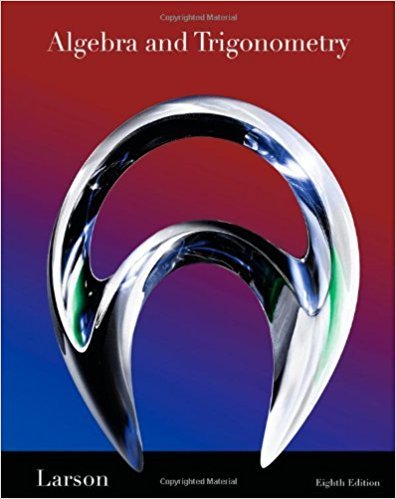×
×

# Solutions for Chapter 1.6: Other Types of Equations## Full solutions for Algebra and Trigonometry | 8th Edition

ISBN: 9781439048474Solutions for Chapter 1.6: Other Types of Equations

Solutions for Chapter 1.6
4 5 0 350 Reviews
11
5
##### ISBN: 9781439048474

Algebra and Trigonometry was written by and is associated to the ISBN: 9781439048474. This textbook survival guide was created for the textbook: Algebra and Trigonometry, edition: 8. This expansive textbook survival guide covers the following chapters and their solutions. Chapter 1.6: Other Types of Equations includes 130 full step-by-step solutions. Since 130 problems in chapter 1.6: Other Types of Equations have been answered, more than 46609 students have viewed full step-by-step solutions from this chapter.

Key Math Terms and definitions covered in this textbook
• Column picture of Ax = b.

The vector b becomes a combination of the columns of A. The system is solvable only when b is in the column space C (A).

• Elimination.

A sequence of row operations that reduces A to an upper triangular U or to the reduced form R = rref(A). Then A = LU with multipliers eO in L, or P A = L U with row exchanges in P, or E A = R with an invertible E.

• Independent vectors VI, .. " vk.

No combination cl VI + ... + qVk = zero vector unless all ci = O. If the v's are the columns of A, the only solution to Ax = 0 is x = o.

• Left nullspace N (AT).

Nullspace of AT = "left nullspace" of A because y T A = OT.

• Lucas numbers

Ln = 2,J, 3, 4, ... satisfy Ln = L n- l +Ln- 2 = A1 +A~, with AI, A2 = (1 ± -/5)/2 from the Fibonacci matrix U~]' Compare Lo = 2 with Fo = O.

• Multiplicities AM and G M.

The algebraic multiplicity A M of A is the number of times A appears as a root of det(A - AI) = O. The geometric multiplicity GM is the number of independent eigenvectors for A (= dimension of the eigenspace).

• Network.

A directed graph that has constants Cl, ... , Cm associated with the edges.

• Normal matrix.

If N NT = NT N, then N has orthonormal (complex) eigenvectors.

• Orthonormal vectors q 1 , ... , q n·

Dot products are q T q j = 0 if i =1= j and q T q i = 1. The matrix Q with these orthonormal columns has Q T Q = I. If m = n then Q T = Q -1 and q 1 ' ... , q n is an orthonormal basis for Rn : every v = L (v T q j )q j •

• Random matrix rand(n) or randn(n).

MATLAB creates a matrix with random entries, uniformly distributed on [0 1] for rand and standard normal distribution for randn.

• Rotation matrix

R = [~ CS ] rotates the plane by () and R- 1 = RT rotates back by -(). Eigenvalues are eiO and e-iO , eigenvectors are (1, ±i). c, s = cos (), sin ().

• Row space C (AT) = all combinations of rows of A.

Column vectors by convention.

• Saddle point of I(x}, ... ,xn ).

A point where the first derivatives of I are zero and the second derivative matrix (a2 II aXi ax j = Hessian matrix) is indefinite.

• Simplex method for linear programming.

The minimum cost vector x * is found by moving from comer to lower cost comer along the edges of the feasible set (where the constraints Ax = b and x > 0 are satisfied). Minimum cost at a comer!

• Singular matrix A.

A square matrix that has no inverse: det(A) = o.

• Singular Value Decomposition

(SVD) A = U:E VT = (orthogonal) ( diag)( orthogonal) First r columns of U and V are orthonormal bases of C (A) and C (AT), AVi = O'iUi with singular value O'i > O. Last columns are orthonormal bases of nullspaces.

• Subspace S of V.

Any vector space inside V, including V and Z = {zero vector only}.

• Symmetric matrix A.

The transpose is AT = A, and aU = a ji. A-I is also symmetric.

• Trace of A

= sum of diagonal entries = sum of eigenvalues of A. Tr AB = Tr BA.

• Vector v in Rn.

Sequence of n real numbers v = (VI, ... , Vn) = point in Rn.

×